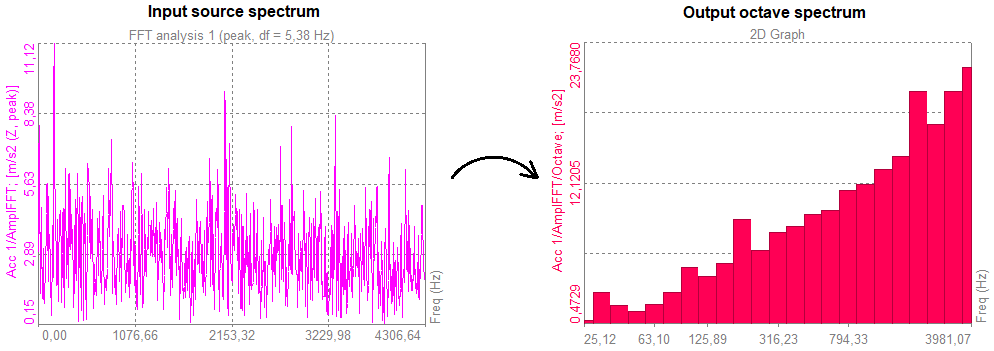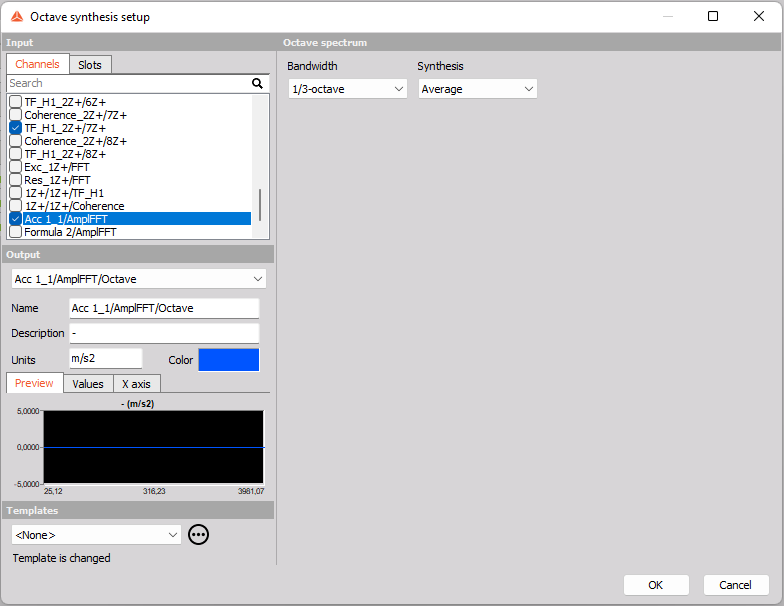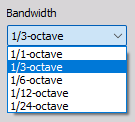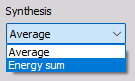# Octave synthesis

The Octave synthesis math module can be used to produce octave spectra from other spectral data.The module requires spectral data as input. Spectral data can e.g. be FFT spectra or modal FRF spectra. Octave spectra have a constant percentage bandwidth, meaning that the spectral bands will increase the bandwidth with a certain percentage as the frequency is increased.
For example, the bandwidth of 1/1-octave bands will increase 100 % per octave (per doubling in frequency) - resulting in bands with bandwidths of e.g. 10 Hz, 20 Hz, 40 Hz, 80 Hz and so on.

Under Octave synthesis setup you can adjust the properties shown below:## Bandwidth

The banwidth parameter determines the relative width of the output spectral bands. The output spectral bands will get more narrow if the fraction of the octave bands are set less e.g. to 1/3-octave from 1/1-octave. For example, using 1/12-octaves will produce spectra having 12 lines or bars per octave, and hereby 12 bands for each time the frequnecy is doubled.NOTE: The lowest band included in the output spectrum will be the lowest band that still has a bandwidth larger than the input spectral line spacing, df.
For example, if the input spectrum has a df = 5 Hz, then the output spectrum will have a lower band at the most narrow band still having a width above 5 Hz.

## Synthesis

The synthesis parameter determines if input data for each 1/n-octave band are averaged together or energy summed.Average - is prefered e.g. when transfer functions has to be decribed with fractional octave bands, since the phase information will be handled properly.

Energy sum - is prefered when you want to produce synthesized octave spectra from e.g. FFT spectra, where the amplitude band values will be similar to results from the Octave analysis math module which use time data as input.